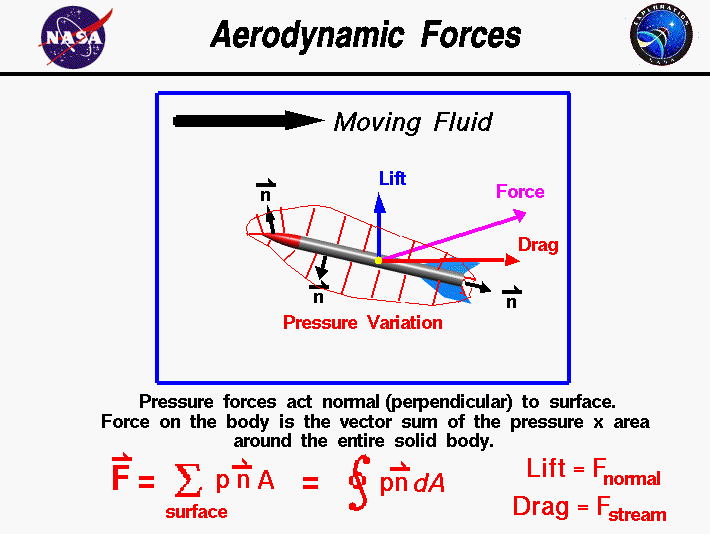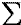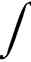+ Text Only Site
+ Non-Flash Version
+ Contact GlennWhen two solid objects interact in a mechanical process, forces are transmitted, or applied, at the point of contact. But when a solid object interacts with a fluid, things are more difficult to describe because the fluid can change its shape. For a solid body immersed in a fluid, the "point of contact" is every point on the surface of the body. The fluid can flow around the body and maintain physical contact at all points. The transmission, or application, of mechanical forces between a solid body and a fluid occurs at every point on the surface of the body. And the transmission occurs through the fluid pressure. Variation in Pressure The magnitude of the force acting over a small section of an object immersed in a fluid equals the pressure p times the area A of the section. A quick units check shows that: p * A = (force/area) * area = force As discussed on the fluid pressure slide, pressure is a scalar quantity related to the momentum of the molecules of a fluid. Since a force is a vector quantity, having both magnitude and direction, we must determine the direction of the force. Pressure acts perpendicular (or normal) to the solid surface of an object. So the direction of the force on the small section of the object is along the normal to the surface. We denote this direction by the letter n. The normal direction changes from the front of the rocket to the rear and from the top to the bottom. To obtain the net mechanical force over the entire solid object, we must sum the contributions from all the small sections. Mathematically, the summation is indicated by the Greek letter sigma () The net aerodynamic force F is equal to the sum of the product of the pressure p times the area A in the normal direction. F =p * A * n In the limit of infinitely small sections, this gives the integral of the pressure times the area around the closed surface. Using the symbolfor integration, we have: F =(p * n) dA where the integral is taken all around the body. On the figure, that is why the integral sign has a circle through it. If the pressure on a closed surface is a constant, there is no net force produced because the summation of the directions of the normal adds up to zero. For every small section there is another small section whose normal points in exactly the opposite direction. F =(p * n) dA = p *n dA = 0 For a fluid in motion, the velocity has different values at different locations around the body. The local pressure is related to the local velocity, so the pressure also varies around the closed surface and a net force is produced. Summing the pressure perpendicular to the surface times the area around the body produces a net force. F =(p * n) dA Definitions of Lift and Drag Since the fluid is in motion, we can define a flow direction along the motion. The component of the net force perpendicular (or normal) to the flow direction is called the lift; the component of the net force along the flow direction is called the drag. These are definitions. In reality, there is a single, net, integrated force caused by the pressure variations along a body. This aerodynamic force acts through the average location of the pressure variation which is called the center of pressure. Velocity Distribution For an ideal fluid with no boundary layers, the surface of an object is a streamline. If the velocity is low, and no energy is added to the flow, we can use Bernoulli's equation along a streamline to determine the pressure distribution for a known velocity distribution. If boundary layers are present, things are a little more confusing, since the external flow responds to the edge of the boundary layer and the pressure on the surface is imposed from the edge of the boundary layer. If the boundary layer separates from the surface, it gets even more confusing. How do we determine the velocity distribution around a body? To correctly determine the velocity distribution, we have to solve equations expressing a conservation of mass, momentum, and energy for the fluid passing the object. In some cases, we can solve simplified versions of the equations to determine the velocity. For coasting rockets, this is particularly difficult because the flow normally separates at the nozzle end of the rocket. To determine the aerodynamic force on the rocket in this situation, we usually build a model of the rocket and measure the aerodynamic force in a wind tunnel. Using the lift and drag equations we can scale the measured forces from the test conditions to flight conditions. Summary To summarize, for any object immersed in a fluid, the mechanical forces are transmitted at every point on the surface of the body. The forces are transmitted through the pressure, which acts perpendicular to the surface. The net force can be found by integrating (or summing) the pressure times the area around the entire surface. For a moving flow, the pressure will vary from point to point because the velocity varies from point to point. For some simple flow problems, we can determine the pressure distribution (and the net force) if we know the velocity distribution by using Bernoulli's equation. For more complex flow problems, we use wind tunnel testing to determine the aerodynamic force. Guided ToursForces on a Rocket:Rocket Aerodynamics:Activities: Related Sites: Rocket Index Rocket Home Beginner's Guide Home+ Inspector General Hotline + Equal Employment Opportunity Data Posted Pursuant to the No Fear Act + Budgets, Strategic Plans and Accountability Reports + Freedom of Information Act + The President's Management Agenda + NASA Privacy Statement, Disclaimer, and Accessibility CertificationEditor: Tom Benson NASA Official: Tom Benson Last Updated: May 13 2021 + Contact Glenn